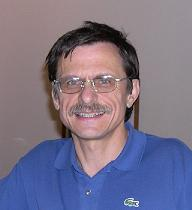# THEMATIC PROGRAMSSeptember 25, 2023THE FIELDS INSTITUTE FOR RESEARCH IN MATHEMATICAL SCIENCES
 January-June 2014 Thematic Program on Abstract Harmonic Analysis, Banach and Operator Algebras Coxeter Lecture Series May 27,28,29, 2014 at 3:30 p.m. Fields Institute, Room 230 Sorin Popa University of California, Los Angeles On $\text{II}_1$ factors arising from free groups acting on spacesThematic Program Home Page Video of the talks will be available at FieldsLive Distinguished and Coxeter LecturersOn $\text{II}_1$ factors arising from free groups acting on spaces A famous problem going back to Murray and von Neumann (1936-1943), asks whether the $\text{II}_1$ factors $L(\Bbb F_n)$, obtained as the centralizers in ${\cal B}(\ell^2 \Gamma)$ of the left regular representation of the free groups on $n$ generators, are non-isomorphic for different $n$. While this is still open, its "group measure space" version, asking whether the crossed product $\text{II}_1$ factors $L^\infty(X)\rtimes \Bbb F_n$, arising from free ergodic probability measure preserving actions $\Bbb F_n\curvearrowright X$, are non-isomoprphic for $n= 2, 3, ...$, independently of the actions, has recently been settled by Stefaan Vaes and myself. I will comment on this result, as well as on some related problems. Speakers in the Distinguished and Coxeter Lecture Series have made outstanding contributions to their field of mathematics. The Lecture Sereies consists of three one-hour lectures.